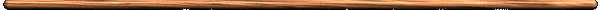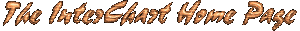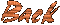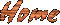Intervals

½ steps Name Spelling Inversion
0 Perfect Unison P1 = 1 P8 = 8
1 minor 2nd m2 = b2 M7 = 7
2 Major 2nd M2 = 2 m7 = b7
3 minor 3rd
Augmented 2nd
m3 = b3
A2 = #2
M6 = 6
d7 = bb7
4 Major 3rd M3 = 3 m6 = b6
5 Perfect 4th P4 = 4 P5 = 5
6 Augmented 4th
diminished 5th
A4 = #4
d5 = b5
d5 = b5
A4 = #4
7 Perfect 5th P5 = 5 P4 = 4
8 Augmented 5th
minor 6th
A5 = #5
m6 = b6
d4 = b4
M3 = 3
9 Major 6th
diminished 7th
M6 = 6
d7 = bb7
m3 = b3
A2 = #2
10 minor 7th m7 = b7 M2 = 2
11 Major 7th M7 = 7 m2 = b2
12 Perfect Octave P8 = 8 P1 = 1